# Boylestad: MCQ in Feedback and Oscillator Circuits

(Last Updated On: December 2, 2019)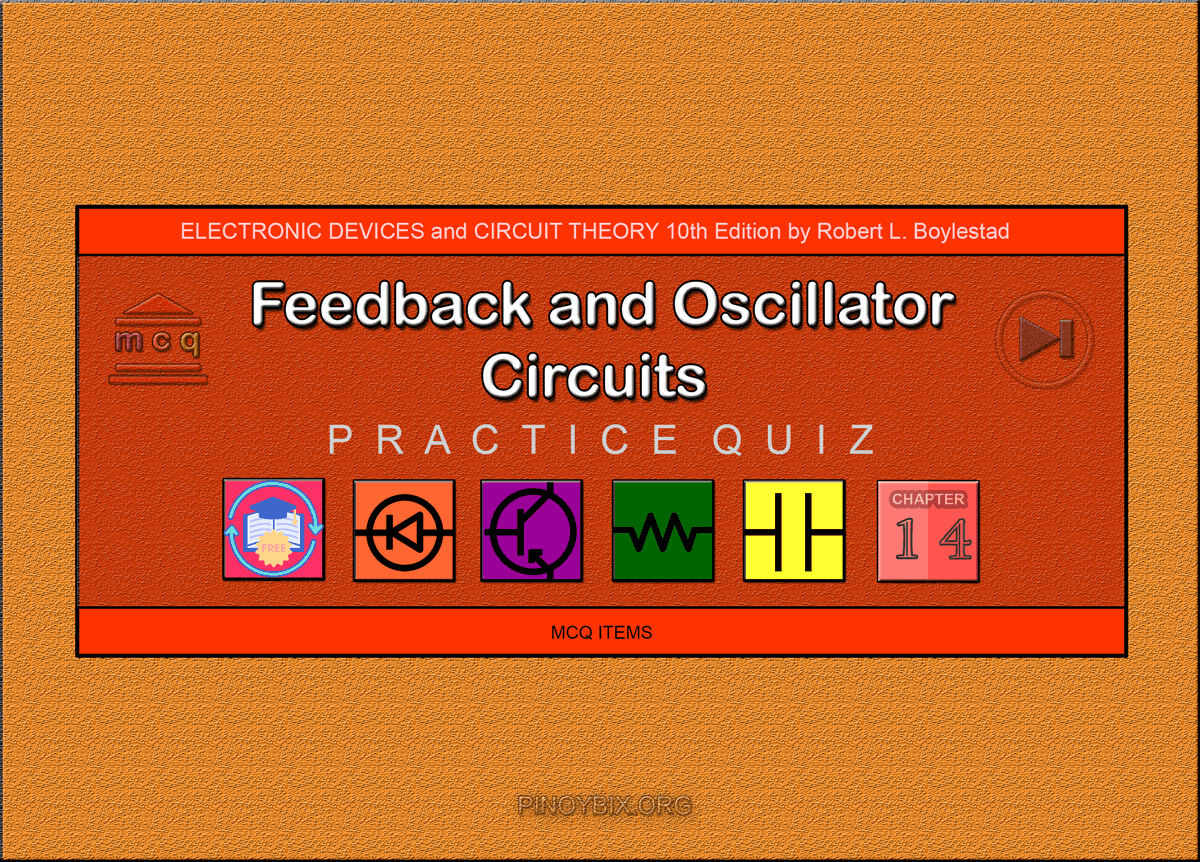This is the Multiple Choice Questions in Feedback and Oscillator Circuits from the book Electronic Devices and Circuit Theory 10th Edition by Robert L. Boylestad. If you are looking for a reviewer in Electronics Engineering this will definitely help. I can assure you that this will be a great help in reviewing the book in preparation for your Board Exam. Make sure to familiarize each and every questions to increase the chance of passing the ECE Board Exam.

#### Online Questions and Answers Topic Outline

• MCQ in Feedback Concepts | MCQ in Feedback Connection Types | MCQ in Practical Feedback Circuits | MCQ in Feedback Amplifier | MCQ in Oscillator Operation | MCQ in Phase-Shift Oscillator | MCQ in Wien Bridge Oscillator | MCQ in Tuned Oscillator Circuit | MCQ in Crystal Oscillator | MCQ in Unijunction Oscillator

#### Practice Exam Test Questions

Choose the letter of the best answer in each questions.

1. Which of the following improvements is (are) a result of the negative feedback in a circuit?

A. Higher input impedance

B. Better stabilized voltage gain

C. Improved frequency response

D. All of the above

Solution:

2. Which of the following improvements is (are) a result of the negative feedback in a circuit?

A. Lower output impedance

B. Reduced noise

C. More linear operation

D. All of the above

Solution:

3. Which of the following is (are) feedback?

A. Voltage-series

B. Voltage-shunt

C. Current-series

D. All of the above

Solution:

4. What is the ratio of the input impedance with series feedback to that without feedback?

A. 1 + βA

B. βA

C. β

D. 1

Solution:

5. What is the ratio of the output impedance with series feedback to that without feedback?

A. 1

B. 1 + βA

C. βA

D. A

Solution:

6. The frequency distortion arising because of varying amplifier gain with frequency is considerably reduced in a negative-voltage feedback amplifier circuit.

A. True

B. False

Solution:

7. Determine the voltage gain with feedback for a voltage-series feedback having A = –100, R1 = 15 kΩ, Ro = 20 kΩ, and a feedback of β = –0.25.

A. 3.85

B. –3.85

C. –9.09

D. 9.09

Solution:

8. Determine the input impedance with feedback for a voltage-series feedback having A = –100, R1 = 15 kΩ, Ro = 20 kΩ, and a feedback of β = –0.25.

A. 110 kΩ

B. 290 kΩ

C. 390 kΩ

D. 510 kΩ

Solution:

9. Determine the output impedance with feedback for a voltage-series feedback having A = –100, R1 = 15 kΩ, Ro = 20 kΩ, and a feedback of β = –0.25.

A. 0.2 kΩ

B. 392.16 kΩ

C. 1.82 kΩ

D. 769.23 Ω

Solution:

10. An amplifier with a gain of –500 and a feedback of β = –0.1 has a gain change of 15% due to temperature. Calculate the change in gain of the feedback amplifier.

A. 0.2%

B. 0.3%

C. 0.4%

D. 0.5%

Solution:

11. Referring to this figure, calculate the amplification gain with feedback for the following circuit values: R1 = 80 kΩ, R2 = 20 kΩ, Ro = 10 kΩ, RD = 61 kΩ, and gm = 4000 μS.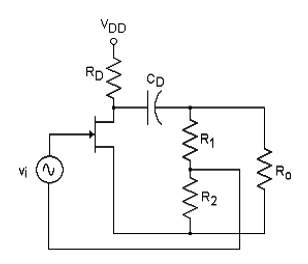A. –4.36

B. –4.25

C. –6.35

D. –20.85

Solution:

12. Referring to this figure, calculate the amplification gain where the op-amp gain (A) is 200,000, R1 = 1.5 kΩ, and R2 = 400 Ω.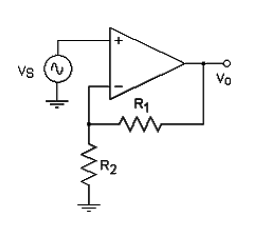A. 4.25

B. 4.50

C. 4.75

D. 5.00

Solution:

13. Referring to this figure, calculate the voltage gain with feedback Avf.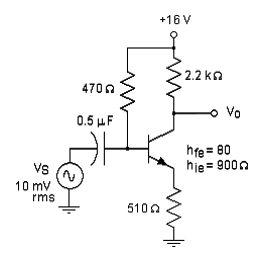A. –4.85

B. –4.20

C. –4.17

D. –4.00

Solution:

14. Referring to this figure, calculate the voltage gain without feedback for the following circuit values: RD = 4 kΩ, RS = 1 kΩ, RF = 15 kΩ, and gm = 5000 μS.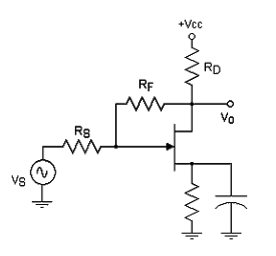A. –20.0

B. –21.5

C. –23.5

D. –25.5

Solution:

15. Referring to this figure, calculate the voltage gain with the feedback for the following circuit values: RD = 4 kΩ, RS = 1 kΩ, RF = 15 kΩ, and gm = 5000 μS.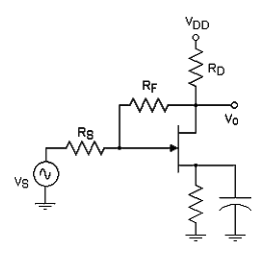A. –11.2

B. –8.57

C. –6.75

D. –3.25

Solution:

16. Which of the following is (are) the determining factor(s) of the stability of a feedback amplifier?

A. A

B. Phase shift between input and output signals

C. Both A and the phase shift between input and output signals

D. None of the above

Solution:

17. At what phase shift is the magnitude of βA at its maximum in the Nyquist plot?

A. 90°

B. 180°

C. 270°

D. 0°

Solution:

18. At what phase shift is the magnitude of βA at its minimum in the Nyquist plot?

A. 90°

B. 180°

C. 270°

D. 0°

Solution:

19. The Nyquist plot combines the two Bode plots of gain versus frequency and phase shift versus frequency on a single plot.

A. True

B. False

Solution:

20. The amplifier is unstable if the Nyquist curve plotted encloses (encircles) the –1 point, and it is stable otherwise.

A. True

B. False

Solution:

21. Which of the following is required for oscillation?

A. βA > 1

B. The phase shift around the feedback network must be 180º.

C. Both βA > 1 and the phase shift around the feedback network must be 180°.

D. None of the above

Solution:

22. An input signal is needed for an oscillator to start.

A. True

B. False

Solution:

23. Only the condition βA = _____ must be satisfied for self-sustained oscillations to result.

A. 0

B. –1

C. 1

D. None of the above

Solution:

24. Given gm = 5000 µS, rd = 40 kΩ, R = 10 kΩ, and A = 35. Determine the value of RD for oscillator operation at 1 kHz.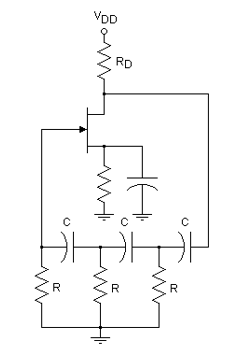A. 8.05 kΩ

B. 8.48 kΩ

C. 10.8 kΩ

D. 12.3 kΩ

Solution:

25. In the IC phase-shift oscillator, what should the ratio of feedback resistor Rf to R1 be?

A. Zero

B. Greater than –29

C. Less than 29

D. Any value

Solution:

26. This circuit is a _______ oscillator.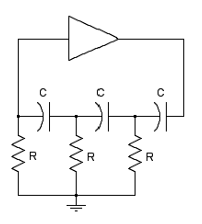A. phase-shift

B. Wien bridge

C. Colpitts

D. Hartley

Solution:

27. For a phase-shift oscillator, the gain of the amplifier stage must be greater than ________.

A. 19

B. 29

C. 30

D. 1

Solution:

28. In the Wien bridge oscillator, which of the following is (are) frequency-determining components?

A. R1 and R2

B. C1 and C2

C. R1, R2, C1, and C2

D. None of the above

Solution:

29. Calculate the resonant frequency of this oscillator.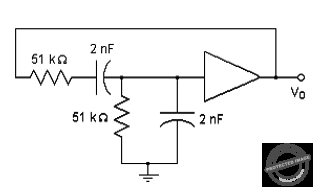A. 1560.34 Hz

B. 3120.70 Hz

C. 4681.07 Hz

D. 6241.37 Hz

Solution:

30. Calculate the resonant frequency of this Wien bridge oscillator if R1 = 25 kΩ, R2 = 40 kΩ, C1 = 0.001 µF, and C2 = 0.002 µF.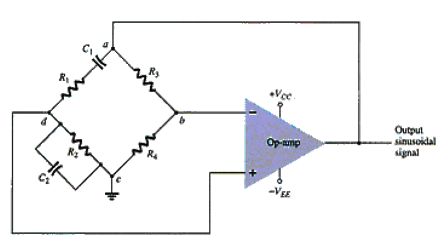A. 1560.3 Hz

B. 1779.4 Hz

C. 3120.7 Hz

D. 3558.8 Hz

Solution:

31. Calculate the value of C1 = C2 for the Wien bridge oscillator to operate at a frequency of 20 kHz. Assume R1 = R2 = 50 kΩ and R3 = 3R4 = 600 Ω?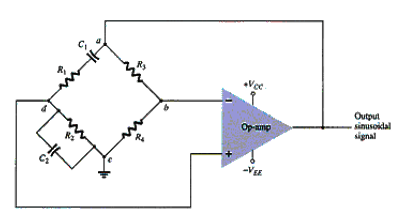A. 1.59 pF

B. 15.9 pF

C. 159 pF

D. 1.59 nF

Solution:

32. This circuit is a ________ oscillator.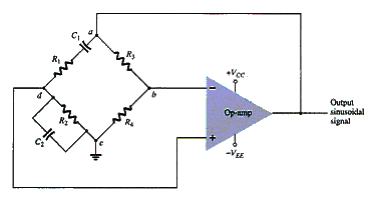A. phase-shift

B. Wien bridge

C. Colpitts

D. Hartley

Solution:

33. Which of the following oscillators is (are) tuned oscillators?

A. Colpitts

B. Hartley

C. Crystal

D. All of the above

Solution:

34. This circuit is a ________ oscillator.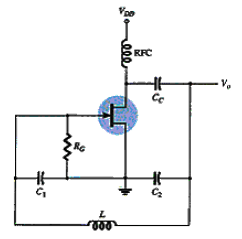A. phase-shift

B. Wien bridge

C. Colpitts

D. Hartley

Solution:

35. What is the typical value of quality factor for crystal oscillators?

A. 20,000

B. 1000

C. 100

D. 10

Solution:

#### FILL-IN-THE-BLANKS

1. Negative feedback results in ________.

A. decreased voltage gain

B increased voltage gain

C. oscillation in the circuit

D. None of the above

Solution:

2. Positive feedback results in _________.

A. decreased voltage gain

B. increased voltage gain

C. oscillation in the circuit

D. None of the above

Solution:

3. Series-feedback connections tend to _______ the input resistance. Shunt feedback connections tend to ________ the input resistance.

A. decrease, increase

B. increase, decrease

C. increase, increase

D. decrease, decrease

Solution:

4. Voltage feedback connections tend to ________ the output impedance. Current feedback connections tend to ________ the output impedance.

A. decrease, increase

B. increase, decrease

C. increase, increase

D. decrease, decrease

Solution:

5. With feedback, β, the overall gain of the circuit is reduced by a factor _______ where A is the gain without the feedback.

A. β

B. Aβ

C. A

D. 1 + βA

Solution:

6. An amplifier with negative feedback has _______ bandwidth than (as) the amplifier without feedback.

A. the same

B. less

C. more

D. None of the above

Solution:

7. A feedback amplifier has a _______ upper 3-dB frequency and a _______ lower 3-dB frequency compared to an amplifier without feedback.

A. smaller, higher

B. higher, smaller

C. smaller, smaller

D. higher, higher

Solution:

8. What is the gain at the origin of the Nyquist plot?

A. Zero

B. A negative value

C. A positive value

D. Undefined

Solution:

9. In a Nyquist plot, as the frequency increases, the phase shift between input and output signals _______.

A. remains the same

B. decreases

C. increases

D. None of the above

Solution:

10. An amplifier is stable if the absolute magnitude of βA is _______.

A. ∞

B. less than 1

C. greater than 1

D. None of the above

Solution:

11. In the Barkhausen criterion, the loop gain A is equal to _______.

A. ∞

B. 200,000

C. 0

D. 1

Solution:

12. In practice, A is made _______ and the system is started oscillating by amplifying noise voltage, which is always present.

A. greater than 1

B. smaller than 1

C. equal to 1

D. None of the above

Solution:

13. In the phase-shift oscillator, the gain of the amplifier stage must be _______.

A. 0

B. less than 29

C. greater than 29

D. ∞

Solution:

14. In the phase-shift oscillator, the operating frequency is determined by _______.

A. resistance only

B. capacitance only

C. LC combinations

D. RC combinations

Solution:

15. In the Wien bridge oscillator with R1 = R2 = R and C1 = C2 = C, a ratio of R3 to R4 will provide sufficient loop gain for the circuit to oscillate.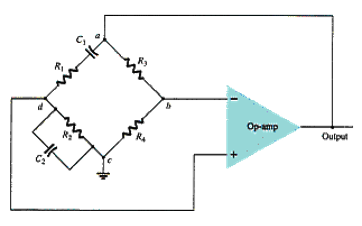A. 0

B. 0.5

C. 1

D. 2

Solution:

16. In the Colpitts oscillator, the frequency is determined by _______ .

A. resistance only

B. inductance only

C. capacitance only

D. both inductance and a capacitance

Solution:

17. In the Colpitts oscillator, the elements X1 and X2 are _______ and X3 is a(n) _______.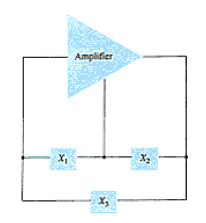A. inductors, capacitor

B. capacitors, inductor

C. capacitors, resistor

D. inductors, resistor

Solution:

18. In a Hartley oscillator, the elements X1 and X2 are _______ and X3 is a(n) _______.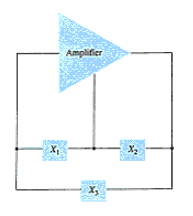A. inductors, capacitor

B. capacitors, inductor

C. capacitors, resistor

D. inductors, resistor

Solution:

19. Crystal oscillators are used whenever a(n) _______ level of stability is required.

A. lower

B. average

C. greater

D. None of the above

Solution:

20. Since the crystal losses represented by R are small, the equivalent crystal Q (quality factor) is _______.

A. very low

B. low

C. medium

D. high

Solution:

21. The series-resonant impedance of a crystal oscillator is _______.

A. very low

B. low

C. medium

D. very high

Solution:

22. The parallel-resonant impedance of a crystal oscillator is _______.

A. very low

B. low

C. medium

D. very high

Solution:

23. At the series-resonant frequency, the amount of positive feedback is _______.

A. very large

B. large

C. small

D. very small

Solution:

24. _______ is a frequency-determining component in a unijunction oscillator.

A. Total resistance

B. Total capacitance

C. Intrinsic stand-off ratio

D. All of the above

Solution:

25. Typically, a unijunction transistor has a stand-off ratio from _____ to _____.

A. 0.0, 0.2

B. 0.2, 0.4

C. 0.4, 0.6

D. 0.6, 0.8

Solution:

### Complete List of Chapter Quiz in Electronic Devices and Circuit Theory

Help Me Makes a Difference!

 P inoyBIX educates thousands of reviewers/students a day in preparation for their board examinations. Also provides professionals with materials for their lectures and practice exams. Help me go forward with the same spirit. “Will you make a small \$5 gift today?” Option 1 : \$1 USD Option 2 : \$3 USD Option 3 : \$5 USD Option 4 : \$10 USD Option 5 : \$25 USD Option 6 : \$50 USD Option 7 : \$100 USD Option 8 : Other Amount1.Unknown
2.dar shahid
3.dar shahid
© 2014 PinoyBIX Engineering. © 2019 All Rights Reserved | How to Donate? |#### GEAS Solution

Dynamics problem Economics problem Physics problem Statics problem Strength problem Thermodynamics problem

#### Questions and Answers in GEAS

Engineering Economics Engineering Laws and Ethics Engineering Management Engineering Materials Engineering Mechanics General Chemistry Giancoli Physics Physics Strength of Materials Thermodynamics
Consider Simple Act of Caring!: LIKE MY FB PAGE

Our app is now available on Google Play, Pinoybix Elex